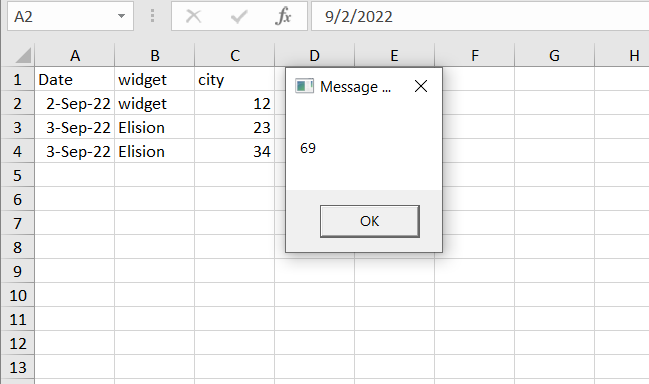# Sum of column data

Hello Robogen,

I want to sum total “Payment Amount” and Total “Amt in Statement” . And want to write both result just after last row dynamically. Could any expert please kindly help

@Sabbir_Anwar Aslam o alaikum
1-Use 2 assign activity.
2-Calculate sum for different columns by using this->
DT.AsEnumerable.Sum(Function(a)Convert.ToDouble(a(“Column A”).ToString))
3-use add datarow activity and give 2 variables to responding column and others will remain empty
like
{“”,“”,TotalPyment,“”,“”,“”,AmtTotal}

Read Range into a datatable then sum the datatable columns.

For how to sum a column, the forum search works great and finds posts like this.

``````(From d in dtData.AsEnumerable Where Not (isNothing(d("Payment Amount")) OrElse String.IsNullorEmpty(d("Payment Amount").toString.Trim)) Select v = CDbl(d("Payment Amount").toString.Trim)).Sum(Function (x) x)
``````

Regards
Gokul

We can do summing up:

Just to name a few options

Check the column name in the excel file @Sabbir_Anwar

Payment Amount

``````(From d in dtData.AsEnumerable Where Not (isNothing(d(4)) OrElse String.IsNullorEmpty(d(4).toString.Trim)) Select v = CDbl(d(4).toString.Trim)).Sum(Function (x) x)
``````

Amt in Statement

``````(From d in dtData.AsEnumerable Where Not (isNothing(d(8)) OrElse String.IsNullorEmpty(d(8).toString.Trim)) Select v = CDbl(d(8).toString.Trim)).Sum(Function (x) x)
``````

Hello @Sabbir_Anwar
Kindly refer to this sample XAML file, you will get some idea

Dtinput.AsEnumerable.Sum(Function(a)Convert.ToDouble(a(“PAYMENT_AMOUNT”).ToString))

@Sabbir_Anwar give me few momentsUpdateColumn.xaml (6.1 KB)

sum is integer

We assume that an assign activity is in use for the Sum LINQ statement
So you can analyze within a debug run the values which are causing issues

• set a breakpoint on the assign activity
• debug and get paused
• open the immediate panel run following statements
• replace 4 with your column index or column name
``````dtData.AsEnumerable.Where(Function (x)  Not (isNothing(d(4) OrElse String.IsNullorEmpty(d(4).toString.Trim))).Select(Function (x) x.ItemArray).toList
``````

now we got all null or empty values

when the result is empty then we check for the non parseable values

``````dtData.AsEnumerable.Where(Function (x)  Not Double.TryParse(d(4).toString.Trim)).Select(Function (x) x.ItemArray).toList
``````

Feel free to share the output with us

Understanding the 6 Debugging Panels of UiPath in the easiest way possible! - News / Tutorials - UiPath Community Forum

Hello @Sabbir_Anwar - Can you share your sample input excel

P_Amount = DT.Asenumerable.Sum(Function(r) CDbl(“0”+r(“Payment Amount”).Tostring.Trim))

A_Amount = DT.Asenumerable.Sum(Function(r) CDbl(“0”+r(“Amt in Statement”).Tostring.Trim))

Now
D_row=DT.Newrow

D_row(“Payment Amount”)=P_Amount

D_row(“Amt in Statemnet”)=A_Amount